# DecayLightFilter

## Overview

The Decay Light Filter causes the light to fade in and fade out. It is a linear ramp multiplier.

## Attribute Reference

Properties attributes

Bool
default: False

Bool
default: False

### far_end

Float
default: 0.0

distance from light to end of fade out

### far_start

Float
default: 0.0

distance from light to start of fade out

### near_end

Float
default: 0.0

distance from light to end of fade in

### near_start

Float
default: 0.0

distance from light to start of fade in

General attributes

### on

Bool
default: True

Turns the light filter on/off.

## Examples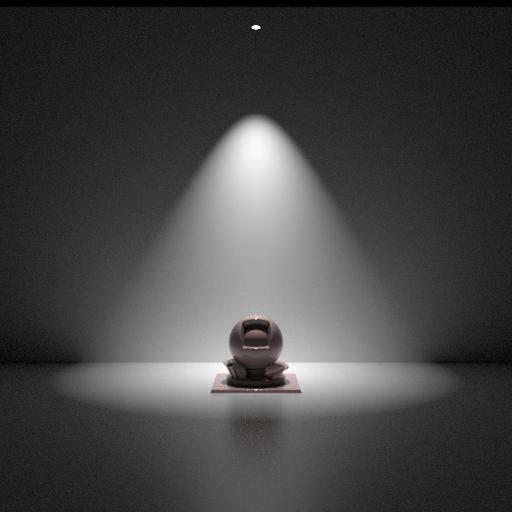No decay
``````filter = DecayLightFilter("/Scene/lighting/decay") {
["falloff near"] = false,
["falloff far"] = false,
}
``````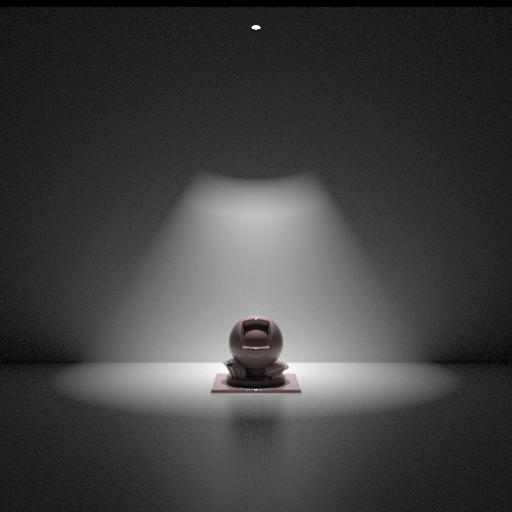``````filter = DecayLightFilter("/Scene/lighting/decay") {
["falloff near"] = true,
["falloff far"] = false,
["near start"] = 4.0,
["near end"] = 5.0,
}
``````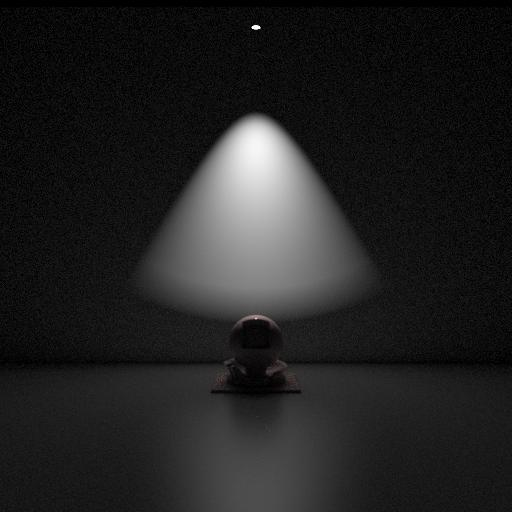``````filter = DecayLightFilter("/Scene/lighting/decay") {
["falloff near"] = false,
["falloff far"] = true,
["far start"] = 7.0,
["far end"] = 8.0,
}
``````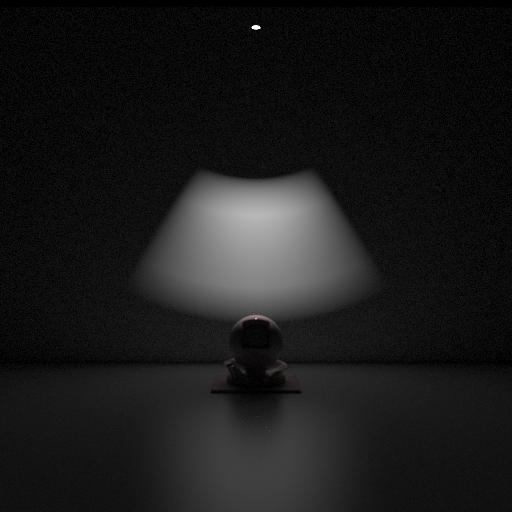``````filter = DecayLightFilter("/Scene/lighting/decay") {
["falloff near"] = true,
["falloff far"] = true,
["near start"] = 4.0,
["near end"] = 5.0,
["far start"] = 7.0,
["far end"] = 8.0,
}
``````Sharp decay
``````filter = DecayLightFilter("/Scene/lighting/decay") {
["falloff near"] = true,
["falloff far"] = true,
["near start"] = 5.0,
["near end"] = 5.0,
["far start"] = 7.6,
["far end"] = 7.6,
}
``````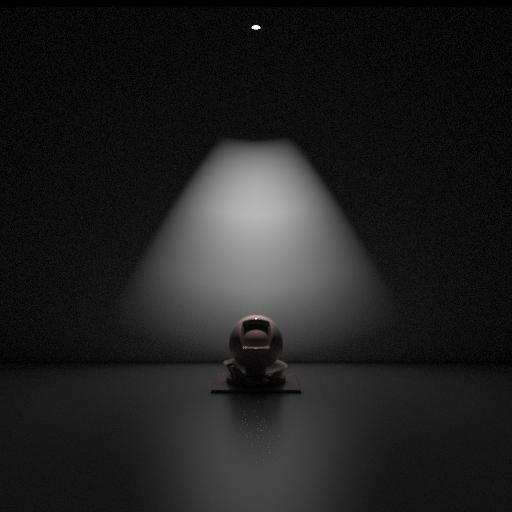``````filter = DecayLightFilter("/Scene/lighting/decay") {